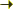Bilbao Crystallographic ServerTensors for different orientation domains Help

## Raman and Hyper-Raman tensors for different orientation domains

 Raman and Hyper-Raman tensors for different orientation domains
 This program provides the Raman or Hyper-Raman tensors for the different orientation domains. Given the high and low symmetry structure and the transformation matrix which relates them the program calculates which are going to be the orientational (twin) domains and finally it gives the tensors for these orientations. If you do not know the transformation matrix you can use the program STRUCTURE RELATIONS. The program only accepts, as input data, structures described in a standard/default setting of the space group. If the original structure is described with respect to an ITA setting, the program SETSTRU can be used to transform it to the standard setting. In the case of more arbitrary non-conventional settings, the tool TRANSTRU can be of some help, if the transformation to the standard setting is known.

1. Select the type of process you want to work with:
Raman tensors
Hyper-Raman tensors

2. Introduce the high and low symmetry structure directly or in CIF format

Structure Data
[in CIF format]

HINT: [ The option for a given filename is preferential ]
High Symmetry Structure
Structure Data
[in CIF format]

HINT: [ The option for a given filename is preferential ]
Low Symmetry Structure

3. Introduce the transformation matrix which relates both structures, you can use STRUCTURE RELATIONS to calculate this matrix. You can introduce in abc form or in matrix form.

In abc form:
In matrix form:
Linear part Origin Shift

[ Bilbao Crystallographic Server Main Menu ]